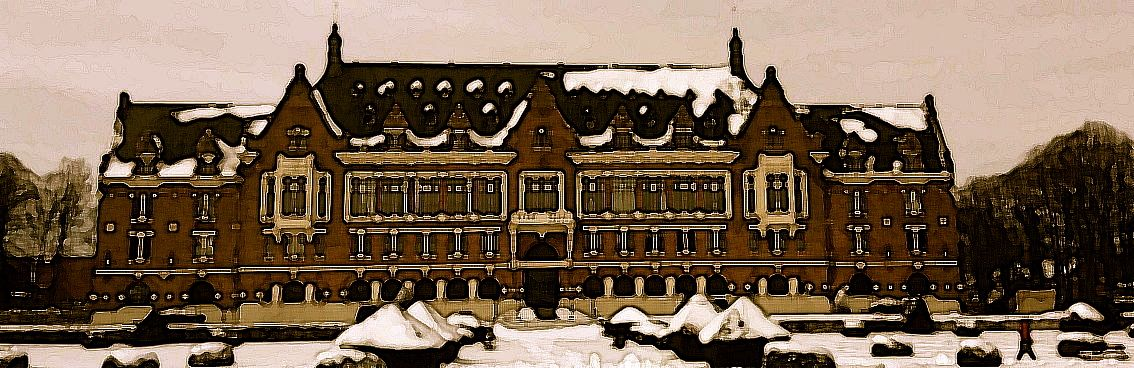# Welcome to the website of the Laboratoire de Mathématiques de Lens

The Laboratoire de Mathématiques de Lens (UR 2462) is part of Université d’Artois. It is a member of the Fédération de Recherche Mathématique des Hauts-de-France FR2037 - CNRS.

It has multiple research areas (clic on the title of each team below to see a more detailed description and the list of members of the team).

Its publications are listed on HAL.

The projects managed or partially managed by the LML are listed on this page.

## Algebra

• Quadratic forms over fields (splitting of bilinear and quadratic forms, characteristic 2)

• Hermitian K-theory, Witt groups of fields and schemes

• Triangulated and stable infinity categories, derived categories

• Homotopy of schemes and cohomological theories (Chow groups, K-théory, algebraic cobordism)

• Noncommutative algebra (ring extensions, noncommutative symmetric functions, Hopf algebras, quantum groups)

## Functional Analysis

• Operator theory

• Geometry of Banach spaces

• Complex Analysis

• Harmonic Analysis

## Geometry

• Algebraic geometry

• Mathematical physics

• Singularities

• Machine learning, deep learning, deep learning of geometry, generative adverserial networks (GANs), distance photoplethysmography

## Didactics of mathematics and Histoiry of mathematics

• Study of professional development of teachers (primary school and highschool)

• Production of material, initial and ongoing training

• Use of history of mathematics in class and in learning

• Study of speach phenomena in teaching and in the learning of mathematics

• History of mathematical theories and concepts (XVIth-XIXth centuries)

• History of mathematical teaching, history in mathematical teaching